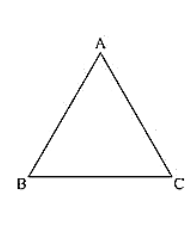# Angles ΔA, B, C of a triangle ABC are equal to each other. Prove that ABC is equilateral.Question:

Angles ΔA, B, C of a triangle ABC are equal to each other. Prove that ABC is equilateral.

Solution:

Given that angles A, B, C of a triangle ABC equal to each other.

We have to prove that ΔABC is equilateral

We have, ∠A = ∠B = ∠C

Now,

∠A = ∠B

BC = AC [opposite sides to equal angles are equal]

And ∠B = ∠C

AC = AB

From the above we get

AB = BC = AC

ΔABC is equilateral.